Courses

# Computer Science And IT (CS/IT) Mock Test 1 For Gate

## 65 Questions MCQ Test GATE Computer Science Engineering(CSE) 2022 Mock Test Series | Computer Science And IT (CS/IT) Mock Test 1 For Gate

Description
This mock test of Computer Science And IT (CS/IT) Mock Test 1 For Gate for GATE helps you for every GATE entrance exam. This contains 65 Multiple Choice Questions for GATE Computer Science And IT (CS/IT) Mock Test 1 For Gate (mcq) to study with solutions a complete question bank. The solved questions answers in this Computer Science And IT (CS/IT) Mock Test 1 For Gate quiz give you a good mix of easy questions and tough questions. GATE students definitely take this Computer Science And IT (CS/IT) Mock Test 1 For Gate exercise for a better result in the exam. You can find other Computer Science And IT (CS/IT) Mock Test 1 For Gate extra questions, long questions & short questions for GATE on EduRev as well by searching above.
QUESTION: 1

### X, Y and Z are the three contestants in one km race. If X can give Y a start of 52 metres and X can also give Z a start of 83 metres, how many metres start Y can give Z?

Solution:

While X runs 1000 metre, Y runs (1000-52) = 948 metre and Z runs (1000-83) = 917 metre
i.e., when Y runs 948 metre, Z runs 917 metre

⇒ When Y runs 1000 metre, Z runs 917/948 x 1000 = 967.30 metre

i.e., Y can give Z (1000-967.30) = 32.7 metre

QUESTION: 2

### There are 5 brothers in a family. All were born at a gap of 3 years. If the sum total of ages of 5 brothers is 100. What is the age of 2nd most elder brother?

Solution:

Let the age of youngest brother be x Then, x + (x + 3) + (x + 6) + (x + 9) + (x + 12) = 100 => 5x = 70 => x = 14 Therefore, age of 2nd most elder brother = 14 + 9 = 23.

QUESTION: 3

### If there are 5 persons who can work individually and complete a work in 2, 4, 5, 6 and 8 hours respectively. If they work together then how much time will it take to finish the work?

Solution:

In 1 hour, ½ + ¼ + ⅕ +⅙ + ⅛ = 149/120 = 1.24 is the work done. Hence, total time to complete the work would be = 1/1.24 = 0.80 hours

QUESTION: 4

If we increase the sides of a rectangular park by 20%, then, what is the total increase in the area of that park ?

Solution:

Let length of park = L and breadth of park = B Original Area = BL m2 New Length = 120L/100 = 6L/5 New Breadth = 120B/100 = 6B/5 New Area = 36LB/25 m2 so, Change in area = New – Original = 11LB/25 m2 % increase = Change / original * 100 = 44%

QUESTION: 5

The sum of the digits in the unit place of all the 4 digit numbers formed with the help of 3, 4, 5 and 6 taken all at a time is:

Solution:

Required sum = 3!(3 + 4 + 5 + 6) = 108 [If we fix 3 of the unit place, other three digits can be arranged in ways similarly for 4, 5, 6.]

QUESTION: 6

Choose the one that best fits the sentence below-

The state’s duty is to _______ the safety of its citizen.

Solution:
QUESTION: 7

Cocaine will have "pernicious" effect on your physical fitness.

Find synonym of the underlined word.

Solution:

Pernicious means harmful effect.

QUESTION: 8

Choose the one that best fits the sentence below.

The _______ of glory lead but to the ______.

Solution:
QUESTION: 9

Gardener said to me, “Don’t pluck the beautiful flowers.”

Select the appropriate Indirect Speech of the above.

Solution:
QUESTION: 10

I haven’t had a call from the office about the meeting ________ last Monday.
Select the appropriate word to fill in the blank from the given options.

Solution:
QUESTION: 11

Let T(n) = 2T(n/4) + 100√n
The value of T(n) can be written as:

Solution: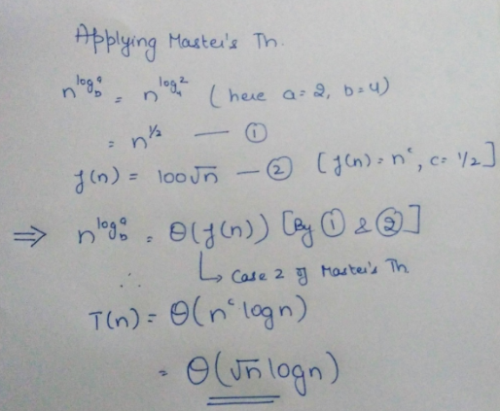QUESTION: 12

Consider a sorted array of n elements. Let k inversions (swapping) have been performed on the sorted array and we want to sort it again to reverse effect of inversions. If K is very small in comparison to n, then which sorting technique will prove efficient for making again the list in sorted increasing order array.

Solution:

Insertion sort is directly proportional to number of inversions present in the list.

QUESTION: 13

What will the output of the following C code?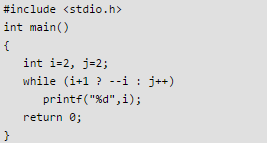Solution:

Consider the while loop condition => i + 1 ? --i : j++ In first iteration : i + 1 = 3 (true) So ternary operator will return --i i.e. 1, condition part is 1 means true so while condition is true. Hence printf statement will print 1 In second iteration : i + 1 =2 (true) So ternary operator will return --i i.e 0, condition part is 0 means false so while condition is false. Hence program control will come out of the while loop.

QUESTION: 14

A balanced tree is given below. How many nodes will become unbalanced when a node is inserted as a child of node G?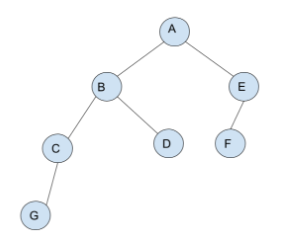Note: A node in a tree is balanced if absolute difference between its left and right subtrees is less than or equal to 1.

Solution: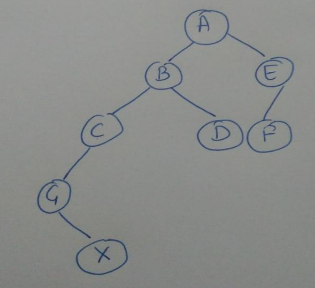In this structure, Node (C) (B) and (A) not having difference between left subtree and right subtree -1, 0, 1. Therefore 3 nodes become unbalanced.

QUESTION: 15

Consider the C code Below.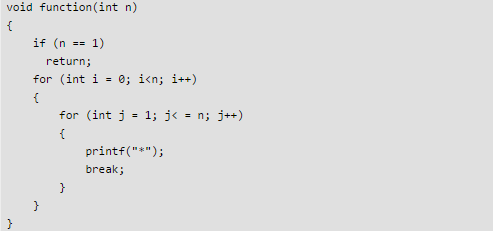Q. Which of the following is the tightest upper bound on time complexity of above function.

Solution:

Important observation is Break statement terminates the innermost loop. So “*” is printed only n times.

QUESTION: 16

Consider the following statements about Bellman ford algorithm for finding shortest path in a directed connected graph G having integral edge weights.

Statement I: It will always find out negative edge weight cycle in G reachable from source.
Statement II: It will always give correct answer for the graph G.

Solution:

Statement 1 is true as in the nth iteration of Bellman Ford, if the length of the path of any node reachable from the source is decreased, that means we are having negative edge weight cycle in the graph. Statement 2 is false as no algorithm can give give correct answer if the Graph is having negative edge weight cycle.

QUESTION: 17

Consider the case: f(n) = O(g(n)). Then, following two statements are claimed to be inferred from the above case.

Statement I: 2f(n) = O(2g(n))
Statement II: 2g(n) = O(2f(n))

Solution:

if f(n) = n and g(n) = 2n. then f(n) = O(g(n)) here, 2^n = O(2^(2n)) = O(4^n), but not vice versa. Hence, I is true. II is false. -------------- Now, if f(n) = 2n and g(n) = n then also f(n) = O(g(n)) because we can ignore constant but, 2^(2n) != O(2^n), hence I is false, but II is true. In both of the above cases, f(n) = O(g(n)). But both the cases are counter of each other. Hence both I and II are wrong.

QUESTION: 18

Let X and Y be the integers representing the number of simple graphs possible with 3 labeled vertices and 3 unlabeled vertices respectively. Let X - Y = N. Then, find the number of spanning trees possible with N labeled vertices complete graph.

Solution:

Number of simple graphs possible with n labeled vertices is 2^(n(n-1)/2). Number of simple graphs possible with n unlabeled vertices is n+1. Number of spanning tree possible with n vertices complete graph n^(n-2) X =8 Y = 4 X-Y=4 Therefore required answer is 4^2=16.

QUESTION: 19

Consider the following pseudo code.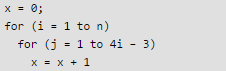Find the value of x, when the above code is executed in a function.

Solution: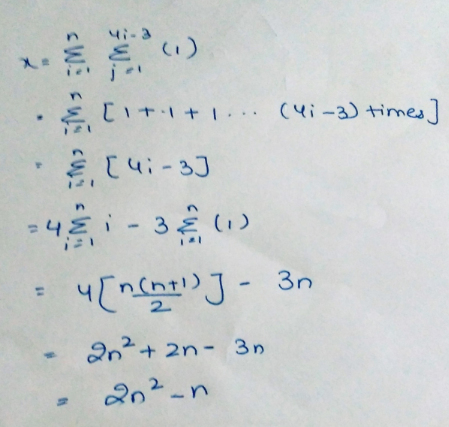QUESTION: 20

Consider the following statements:

S1 : DFS of a directed graph always produces the same number of edges in the traversal, irrespective of the starting vertex.
S2 : If all of the back edges that are found while DFS traversal on directed graph are removed, the resulting graph is acyclic.
Q. Which of the following statements above are valid?

Solution:

Statement S1 : consider the graph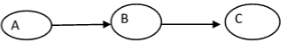Starting with A (source vertex ) we will get 2 edges Starting with B will get only 1 edge Starting with C we will get no edge Therefore DFS on directed graph may not give same number of edges. Statement S2 : Back edges are those edges (u,v) connecting a vertex u to an ancestor u in a depth-first tree. Self-loops are considered to be back edges. Back edges describe descendant-to-ancestor relations, as they lead from “high” to “low” nodes. Suppose that there is a back edge (u, v). Then vertex v is an ancestor of vertex u in the depth-first forest. There is thus a path from v to u in G, and the back edge (u,v) completes a cycle. Removing the back edge will break the cycle. Therefore removing all the back edges will make the graph acyclic. So the statement is true.

QUESTION: 21

Which data structure would be the most appropriate to implement a collection of values with the following three characteristics?

i) Items are retrieved and removed from the collection in FIFO order.
ii) There is no priori limit to the number of items in the collection.
iii) The size of an item is large relative to storage required for a memory address.

Solution:

Head and tail pointers in singly link list will make the insertion and deletion in O(1) time complexity if we are accessing the elements in FIFO order. In doubly link list since only head pointer is given then for insertion we have to traverse the complete link list so insertion will be O(n) so not appropriate. In binary tree we have only a pointer to the root. Insertion and deletion in binary tree will be O(log n) so not appropriate. In hash table accessing the data in FIFO order will not be possible.

QUESTION: 22

Stack A has the entries as following sequence a, b, c (with ‘a’ on top), stack B is empty, as shown in the diagram below.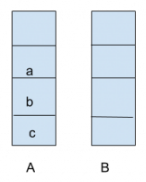Q. An entry popped out of stack A can be printed or pushed to stack B. An entry popped out of stack B can only be printed. In this arrangement which of the following permutation of a, b, c are not possible to print?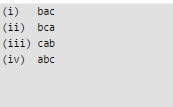Solution:

Follow these steps to print bac.
1) POP element ‘a’ from stack A, push ‘a’ to Stack B.
2) POP element ‘b’ from A, print it.
3) POP element ‘a’ from B, and print it.
4) POP element ‘c’ from A, and print it. Now, perumtation bac has been printed. Similarly, we can print bca and abc, but can’t print cab.

QUESTION: 23

Stack A has the entries as following sequence a, b, c (with ‘a’ on top), Stack B is empty, as shown in the diagram below.Q. Considering the data given in the previous question, if the stack A had 4 entries, then the number of possible permutations that can be printed will be:

Solution: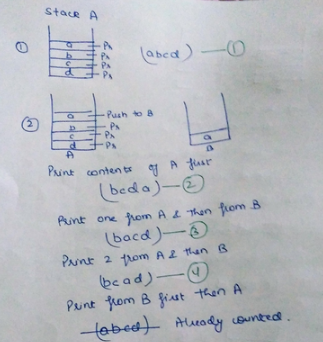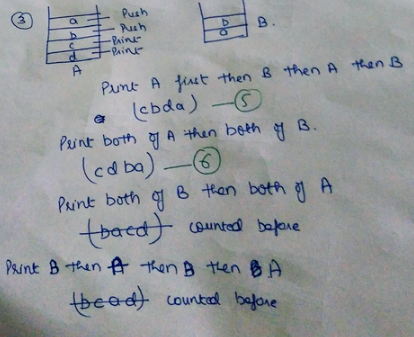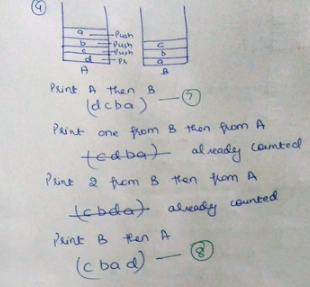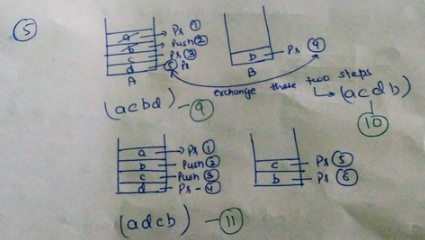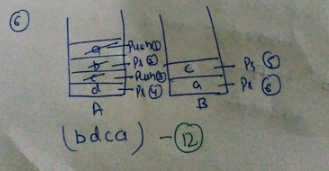QUESTION: 24

Given a hash table with n keys and m slots with simple uniform hashing. If collisions are resolved by chaining then what is the probability that the first slot ends up empty?

Solution:

Probability of one particular slot = 1/m ( because total m slots) Probability that a value should not go in one particular slot = 1 – (1/m) For n values (keys) probability = [1 – (1/m)]n

QUESTION: 25

Consider the following instance of knapsack problem: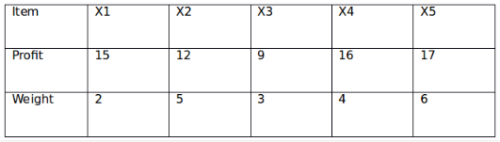Q. The maximum weight of 12 is allowed in the knapsack. Find the value of maximum profit with the optimal solution of the fractional knapsack problem.

Solution: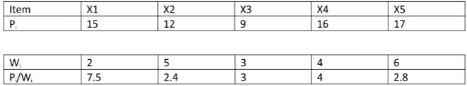QUESTION: 26

Find the maximum value of the expression (x+y+k) where (x,y) satisfies the equation (x-2)2 + (y-3)2= 25

Solution:

Since (X,Y) is a point on circle, the general form of the point is X = 2 + 5*cost, y = 3 + 5*sint We need to maximise the value of x+y+k x+y+k = 2 + 5*cost + 3 + 5*sint + k = (5+k) + 5*(cost+sint) Here, k is a constant. The maximum value of c + acost + bsint is equals to c + sqrt (a*a +b*b). Maximum value of (5+k) + 5*(cost+sint) (5+k) + 5*sqrt(2) The result is (5+k) + 5*sqrt(2).

QUESTION: 27

Which of the following argument is invalid?

Solution:

“⟺” mean equal. ifA⟺B means A is equal to B.
Option (a); (p→(q⋁r) )⟺(~p⋁(q⋁r) ) ⟺((~p⋁q)⋁r) ⟺(~(p⋀~q)⋁r) [∵De morgan’s law] ⟺(p⋀~q)→r ∴(p→(q⋁r) )⟺((p⋀~q)→r)

Option (b); (p→r)⋀(q→r)⟺(~p⋁r)⋀(~q⋁r) ⟺(~p⋀~q)⋁r [∵ De morgan’s law] ⟺~(p⋁q)⋁r ⟺(p⋁q)→r ∴(p→r)⋀(q→r)⟺(p⋁q)→r

Option (c): p→(q→r)⟺~p⋁(q→r) ⟺ ~p⋁(~q⋁r) ⟺~q⋁(~p⋁r) ⟺~q⋁(p→r) ⟺q→(p→r)

Option (d): (p↔q)⟺(p→q)⋀(q→p) ⟺(~p⋁q)⋀(~q⋁p) ⟺(~p⋁q)⋀(p⋁~q) ⟺(~p⋀~q)⋁(p⋀q) ⟺ ~(p⋁q)⋁(p⋀q) (p↔q)⟺(p⋁q)⋁(p⋀q) is wrong.

QUESTION: 28

The value of the following Integral is: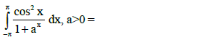Solution: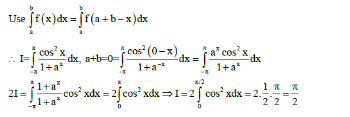QUESTION: 29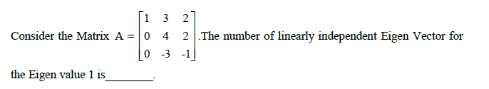Solution: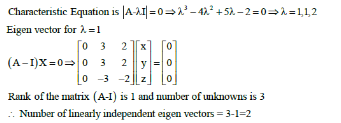QUESTION: 30

Which Of the following statements are true?
S1: Every Distributive lattice has unique complement.
S2: In Complemented lattice every element has at least one complement.
S3: Boolean lattice is both complemented and distributive.
S4: In Boolean lattice every lattice has exactly one complement.

Solution:

Clearly S1 is wrong because in distributive lattice a element may have zero complement. Rest other statements are properties of the corresponding lattices.

QUESTION: 31

Given a graph G (V, E) is Bipartite, what is the chromatic number of G?

Solution:

Since the graph G is bipartite, the vertices set V can be partitioned into two disjoint sets. This shows that we can color the graph with 2 colors such that no two adjacent vertices will have same color.

QUESTION: 32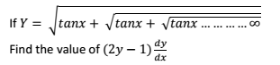Solution: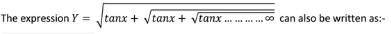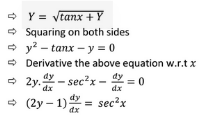QUESTION: 33

Let f(n) = Σ [(log(n/2i) +100] where i limits from 0 to k, and n = 2k.
Find the time complexity of f(n).

Solution: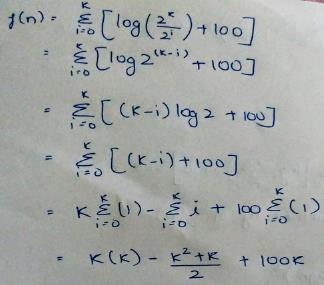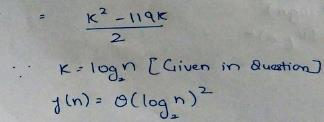QUESTION: 34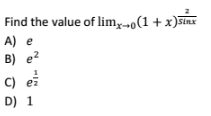Solution: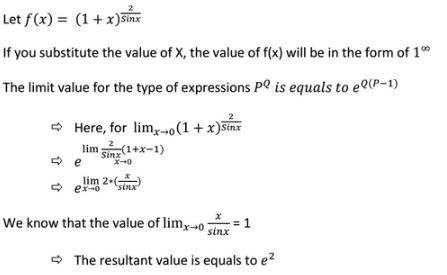QUESTION: 35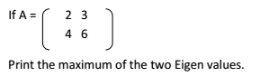Solution: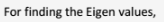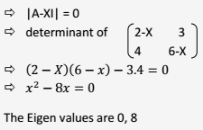QUESTION: 36

If P, Q are the roots of the quadratic equation x2 + ax + b. Find the value of P3 +Q3.

Solution: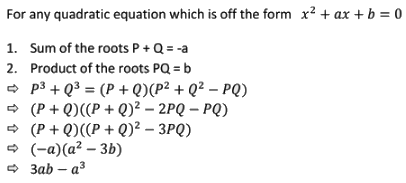QUESTION: 37

Given F is an expression of P,Q. Derive the expression F(P,Q) from the truth table given below.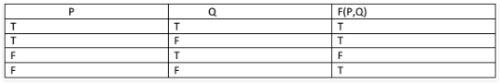Solution: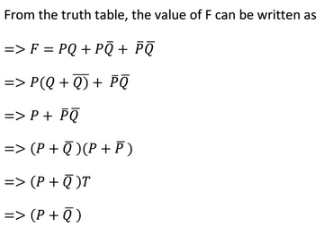QUESTION: 38

In a random experiment, suppose 3 unbiased coins are tossed simultaneously, what is the probability of getting at least 2 Heads?

Solution: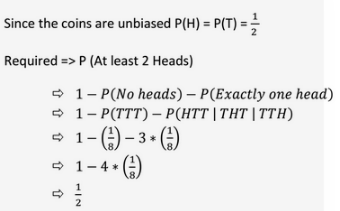QUESTION: 39

Consider a DFA accepting all strings over {a, b} where the number of a’s mod 3 = 2 and number of b’s are odd. What is the minimum number of states of such DFA?

Solution:

The above question is an example of product automata. If we have two cases for which we can have separate DFA’s we can merge the two by product automata. The resulting DFA has number of states equal to the product of the states of the separate DFA’s. Here DFA for accepting all strings over {a, b} where the number of a’s mod 3 = 2 would have 3 states. ( number of a’s mod 3 would give remainder either 0, 1, 2 so 3 states to depict each). Similarly DFA accepting all strings over {a, b} where the number of b’s are odd ( number of b’s mod 2 = 0) would have 2 states. Hence resulting DFA for both the conditions would have 2*3 = 6 states.

QUESTION: 40

Which of the following languages are regular over ∑ = {a, b}?
L1 = { anbn | 0 < n ≤ 10}
L2 = { a2n | n ≥ 1}
L3 = { an! | n > 0}

Solution:

Any language for which we have a finite set or we can possibly have a DFA drawn for it is regular. L1: It gives a finite set generating string with equal number of a’s and equal number of b’s for range 0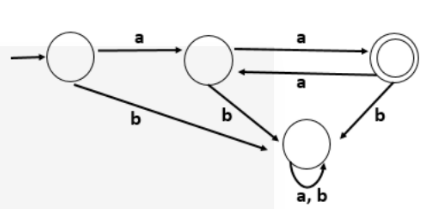Hence L2 is also regular. L3: It gives all possible strings with a’s such that number of a’s are in factorial values of 1,2,3,4 ……. i.e. {a1, a2, a6, a24 ……………}. The set is neither finite nor a DFA is possible for such set. Hence L3 is not regular.

QUESTION: 41

The language L = {WbcWR | W ∈ (a+b)*} is _____.

Solution:

Any language for which we can have a Deterministic PDA is always a DCFL. Here for language L= {WbcWR | W ∈ (a+b)*} we can have a PDA with following transitions in which PDA accepts a string when it halts on a final state. q0 in initial state and qf is final state. 1. (q0, a , Z) -> (q0, aZ) 2. (q0, b , Z) -> (q0, bZ) 3. (q0, a , a) ->(q0, aa) 4. (q0, b , a) -> (q0, ba) 5. (q0, a, b) -> (q0, ab) 6. (q0, b , b) -> (q0, bb) 7. (q0, c , b) -> (q1, null) 8. (q1, a , a) ->(q1, null) 9. (q1, b , b) -> (q1, null) 10. (q1, null, Z ) -> (qf, Z) Here all a’s and b’s are initially pushed onto the stack for W. As soon as a c occurs after b , B is popped from the stack after which W^R is checked. If the further string alphabets match the alphabet of the stack the alphabet is popped from the stack and finally the string reaches the final state if language is of the form L= {WbcW^R | W ∈ (a+b)*}. Hence the above language is a DCFL.

QUESTION: 42

Which of the following languages are closed under complementation?
A) Context free
B) Recursive
C) Recursive Enumerable

Solution:

According to closure properties of languages, context free and recursive enumerable languages are not closed under complementation while recursive language is.

QUESTION: 43

Which of the following statements is incorrect?

Solution:

Statement 1 is correct because union of two DCFL may or may not be DCFL but surely CFL. Statement 2 is correct because regular languages are closed under complementation. Statement 3 is correct because Turing recognizable languages or recursive enumerable languages are closed under intersection.

QUESTION: 44

Which of the following is not a CFL?
A)L = {albmcn where l=m or l=n}
B)L = {albmcn where l=m and l=n}
C)L = {albmcn where l = m + n}

Solution:

Language in A is CFL because CFL can make one comparison which is either l and m must be equal or l and n must be equal. Similarly language in C is also CFL. But B is not because it has to make two comparisons. So language in B is CSL not CFL.

QUESTION: 45

Identify the circuit shown below: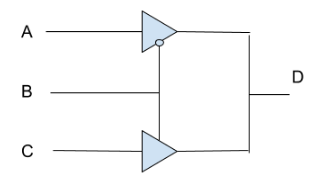Solution:

In the circuit shown there are two tristate buffers. One with active low enable and second one with active high enable. As per the operation of these buffers truth table is given below. It is equivalent to 2 x 1 MUX B is selection line, A & C are the input lines, ‘D’ is the output.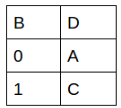QUESTION: 46

The 32 bit floating point representation of (-12) is ___

Solution:

To convert the floating point into decimal, we have 3 elements in a 32-bit floating point representation:

• Sign (MSB)
• Exponent (8 bits after MSB)
• Mantissa (Remaining 23 bits)

Sign bit is the first bit of the binary representation. '1' implies negative number and '0' implies positive number. Sign bit=1 Exponent is decided by the next 8 bits of binary representation. Hence the exponent of 2 will be 3. i.e 23=8. 127 is the unique number for 32 bit floating point representation. It is known as bias. It is determined by 2k-1-1 where 'k' is the number of bits in exponent field. Thus bias = 127 for 32 bit. (28-1-1 = 128-1=127) 127+3=130 i.e. 10000010 in binary representation. Mantissa: 12 in binary = 1100 Move the binary point so that there is only one bit from the left. Adjust the exponent of 2 so that the value does not change. This is normalizing the number. 1.100 x 23 10000000000000000000000 Thus the floating point representation of -12 is 1 10000010 10000000000000000000000

QUESTION: 47

The following is the AB flip flop: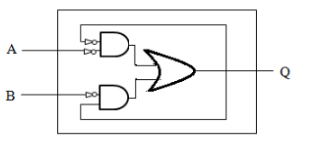What are values of A, B when change in the flip flop state is from 0 to 1?

Note: x in the options below represents that it can have any value out of 0 and 1.

Solution:
QUESTION: 48

An instruction pipeline has 4 stages Instruction Fetch(IF), Instruction Decode(ID), Execute instruction (Ex), Write Back(WB). All instructions take all stages and takes 4 clock cycles. Branch instructions are not overlapped, i.e. the instructions after the branch are not fetched till branch is known. Branch is known in the execute phase. Suppose 20% instructions are conditional and 80 % unconditional. Calculate speed up for 100 instructions (upto 2 decimal place). Ignore the case that the branch may not be taken.

Solution:

Suppose each stage takes 1 s. 20 conditional – 20*3 s (Cycles per instruction for conditional instructions is 3 as branch is known at the third stage) 80 unconditional – 80 s (Cycles per instruction for unconditional is 1) So total time taken with pipeline = 20*3 + 80 = 140 s Time taken without pipeline = 4 * 100 (Cycle per instruction for all is 4) Speed up = 400 / 140 = 2.86

QUESTION: 49

A digital computer has a memory unit of 256k x 16 and a cache memory of 4k words. The cache uses direct mapping with a block size of 16 words.

Q. How many bits are there in index, tag, block and words fields of address format?

Solution:

Main Memory has 256k = 28 x 210 = 218.
i.e. We need 18 bits to address main memory.
Cache Memory has 4k = 22 x 210 = 212.
i.e. We need 12 bits to address cache memory.

Cache consists of Index and tag which together are used to address main memory location.
Here Index is 12 bits and tag is 6 bits. (18 – 12 = 6).

Index is divided into block part and word part. Block part is used to address blocks in cache and word part addresses individual word in a block.

Here a block size is 16 words. i.e. 24, we need 4 bits to address a word and 12 – 4 = 8 bits to address a block in cache memory.

QUESTION: 50

A digital computer has a memory unit of 256k x 16 and a cache memory of 4k words. The cache uses direct mapping with a block size of 16 words.

Q. How many blocks can cache accommodate?

Solution:

Since cache is 4k words and block size is 16 words i.e. No of blocks in cache = 4k/16 = 256.

QUESTION: 51

On a system using round-robin scheduling let 's' represent the time needed to perform a process switch, 'q' represents the round-robin time quantum, and 'r' represents the average time a process runs before blocking on I/O. The CPU efficiency when s = q < r is:

Solution:

‘r’ is the average time a process runs before I/O block and ‘s’ is the time needed for switch. 'q' represents the round-robin time quantum. The efficiency of the CPU when s < r and q < r. = r/(r/q)*s+r = q/(q+s) Here s = q. Hence efficiency is ½.

QUESTION: 52

A counting semaphore was initialized to 0, then 20 V operations were successfully completed on this semaphore, followed with 18 P operations, the resulting value of the semaphore is:

Solution:

20V => increments the semaphore 20 times. Hence , the value becomes 20. 18P => decrements the semaphore 18 times. Hence , the value becomes 2.

QUESTION: 53

Consider all the processes are arriving at large time intervals. Let t be the time interval between two processes Pi and Pi+1 for any i and service time of Pi is Si. If t > Si for every i then, what should be the strategy to schedule the processes?

Solution: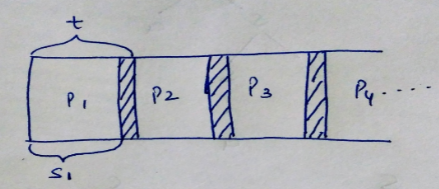If t>Si then all the processes are serviced in FCFS based because when the next process arrives the previous process is already completed.

QUESTION: 54

The following graph shows the schedule of five transactions. The schedule is: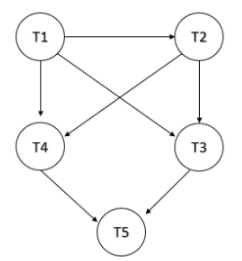Solution:
QUESTION: 55

A student relation with 4 attributes is defines as R(ABCD). Which of the following query will execute without error if all attributes are numeric.
A) ∏B,CA>20)
B) σA>20(∏B,C)

Solution:

Query in A is correct It will first select required rows and then project required columns. But query in B is not correct as after projecting B and C columns. It can't have row selection based on condition on attribute A So, correct option is 1.

QUESTION: 56

Using the EMPLOYEE table, the following query is issued to generate the name, salary and the salary increased after an appraisal by 25 %. The increased salary for all the employee should be above 25000.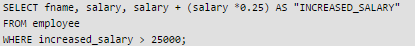Q. The above query throws an error. What is the reason for the error?

Solution:

A column alias cannot be used in WHERE clause conditions but can be used in SELECT statement and ORDER BY clause.

QUESTION: 57

Consider the following CFG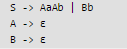Q. The above grammar is:

Solution:

The grammar is unambiguous (only one parse tree) , not left recursive ( Non-terminal not present in second and third grammar rule) . It is left factored . It is LL(1).

QUESTION: 58

Consider the following SDTQ. What will be output by the SDT for the input string “14122323”?

Solution: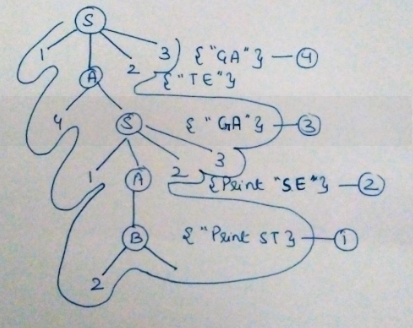QUESTION: 59

A directed acyclic graph represents one form of intermediate representation. The number of non terminal nodes in DAG of a = (b+c)*(b+c) expression is:

Solution: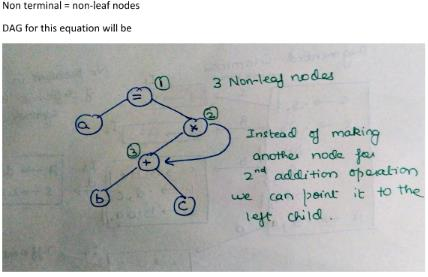QUESTION: 60

Consider the following grammar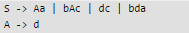Q. The above grammar is:

Solution:
QUESTION: 61

Let 5 stations are connected to CSMA/CD network . Every station wants to transmit data with probability p = 0.6. It is given that there will be no collision if only one station will transmit. What is the probability of successful transmission?

Solution:

Success will be for one station and failure for other four Thus Psucc =5 c1 *(0.6)*(o.4)4 =0.0768.

QUESTION: 62

Consider the following two statements:

Statement 1: Stop and Wait protocol is preferred for LANs compared to WANs.

Statement 2: Stop and Wait protocol is good for bursty data transmission.

Q. Which of the above two statements are true?

Solution:

Efficiency of Stop and Wait is given by, E= 1/(1 +(2*d*B)/v*L), link speed(v) and Bandwith (B) are fixed . Thus only variable terms are packet length(L) and distance(d) Cleary, E decreases with increase in d . And E will increase with increase in L . Hence Stop and Wait is suitable for LANs and bursty data.

QUESTION: 63

A broadcast channel has 10 nodes and total capacity of 10 Mbps. It uses polling for medium access. Once a node finishes transmission, there is a polling delay of 80 μs to poll the next node. Whenever a node is polled, it is allowed to transmit a maximum of 1000 bytes. The maximum throughput of the broadcast channel is

Solution: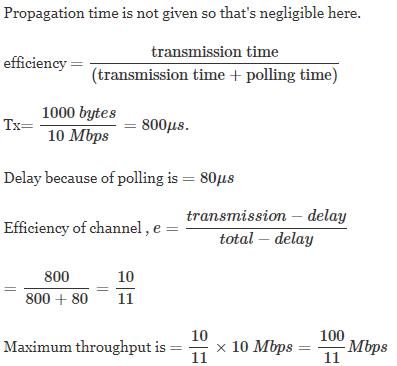QUESTION: 64

Match the protocol with the characteristics:
1. Mails are stored on the computer client use.
2. Stateless protocol.
3. Converts MAC address to IP address.
4. Used to send error messages.

Solution:

In POP3 protocol mails are stored on client’s computer while in IMAP mails are stored on the server. HTTP is a stateless protocol, RARP is used to convert MAC to IP while ARP is used to convert IP to MAC, ICMP is used for sending error messages.

QUESTION: 65

In public key and private key cryptography, host ‘A’ has PuA and PrA, host ‘B’ has PuB and PrB as public and private keys respectively. Now, if ‘A’ wants to send a message to ‘B’ securely, which key will be used by ‘A’ for encryption?

Solution:

If the message is encrypted using PuB, then it can be decrypted using only PrB, which will be known only to ‘B’.